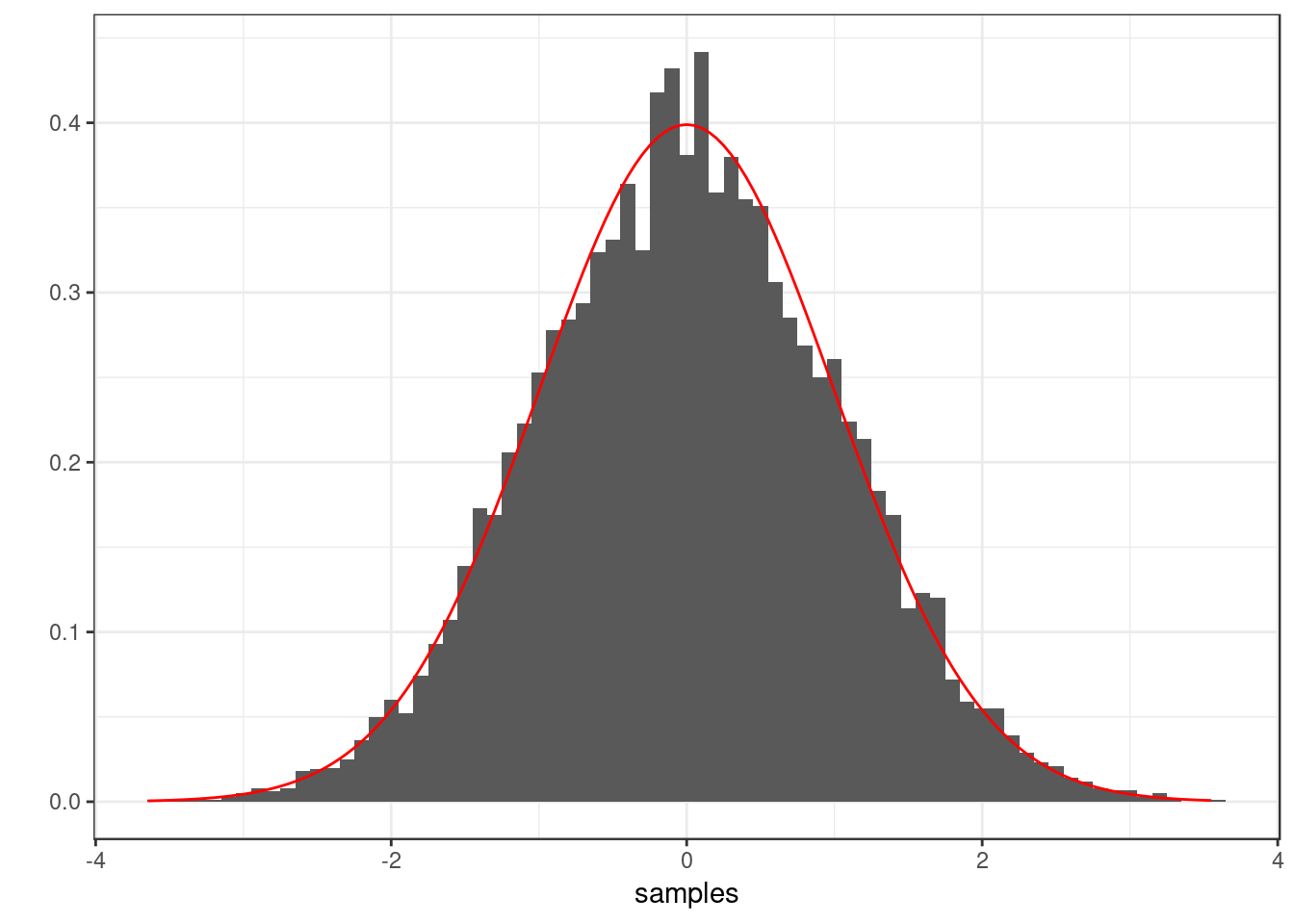### Box-Muller algorithm

As described in Lecture 2.

box_muller <- function(size) {
# Since Box-Muller generates variates in pairs, we round up from the
# requested size.
num_pairs <- ceiling(size/2)

R <- sqrt(-2 * log(runif(num_pairs)))
theta <- 2 * pi * runif(num_pairs)

X <- R * cos(theta)
Y <- R * sin(theta)

c(X, Y)[0:size]
}

Let’s test the function. First, run it.

samples <- box_muller(10001)

Do we have the right number of samples?

cat("length(samples): ", length(samples))
## length(samples):  10001

Plot a histogram of the samples.

library(ggplot2)
theme_set(theme_bw())
graph_hist <- qplot(x = samples, geom = "blank") + geom_histogram(aes(y = ..density..),
binwidth = 0.1)

# Superimpose a line showing the correct density.
xs <- seq(min(samples), max(samples), 0.05)
ys <- dnorm(xs)
df <- data.frame(xs, ys)
graph_hist <- graph_hist + geom_line(aes(x = xs, y = ys), df, colour = "red")
graph_histFinally, perform a Kolmogorov-Smirnov test.

ks.test(samples, "pnorm")
##
##  One-sample Kolmogorov-Smirnov test
##
## data:  samples
## D = 0.011392, p-value = 0.1491
## alternative hypothesis: two-sided